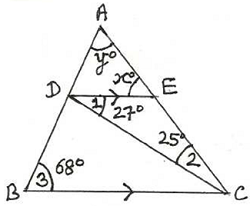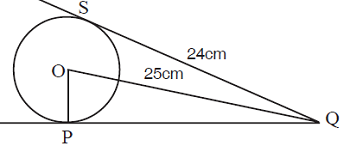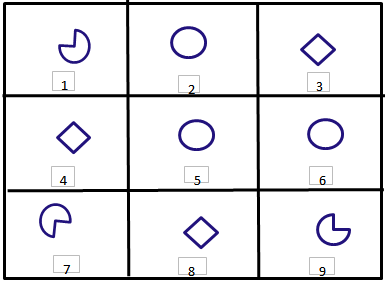# SilverZone - IOM PDF Sample Papers for Class 9

Class 9 sample paper & practice questions for International Olympiad of Mathematics (IOM) level 1 are given below. Syllabus for level 1 is also mentioned for these exams. You can refer these sample paper & quiz for preparing for the exam.#### Resources:

##### Sample Questions from Olympiad Success:
 Q.1 Q.2 Q.3 Q.4 Q.5 Q.6 Q.7 Q.8 Q.9 Q.10
 Q.1 What is the relation between me and my paternal grandfather's son-in-law? a) brother b) maternal uncle c) maternal grandfather d) paternal uncle
 Q.2 Find the value of y if the points A(5, y), B(1, 5), C(2, 1) and D(6, 2) are the vertices of the square. a) 5 b) 3 c) 6 d) 4
 Q.3 If a cuboid is 2.7 cm high, 7.8 cm long and 3.7 cm wide then volume of cuboid is? a) 77.922 cm3 b) 82.987 cm3 c) 83.456 m3 d) 85.687 cm3
 Q.4 Find x and y in the following figure:a) 38⁰, 52⁰ b) 20⁰, 60⁰ c) 52⁰, 60⁰ d) None of these
 Q.5 Look at the image given below and find OP.a) 5 cm b) 6 cm c) 7 cm d) 4 cm
 Q.6 The mean of 5 numbers is 18. If one number is excluded their mean is 16. Find the excluded number. a) 25 b) 26 c) 27 d) 28
 Q.7 The value of polynomial P(x) = x² + 4x - 10 at x = 2 is: a) -2 b) 2 c) -4 d) 4
 Q.8 Identify the identical figures from the following options.a) (1,7,9), (2,5,6), (3,4,8) b) (1,5,9), (2,6,7), (3,4,8) c) (1,7,8), (2,5,6), (3,4,9) d) (1,2,9), (5,6,7), 3,4,8
 Q.9 What is the probability of getting a total of less than 12 in the throws of two dice? a) 35/36 b) 21/36 c) 2/6 d) 3/8
 Q.10 Find the equation of the straight line joining the points of intersection of 2x + y = 4 with x - y + 1 = 0 and 2x - y - 1 = 0 with x + y - 8 = 0. a) x + 2y = 3 b) x + 2y + 3 = 0 c) x - 2y + 3 = 0 d) 3x - 2y + 1 = 0Sample PDF of SilverZone - International Olympiad of Mathematics (IOM) PDF Sample Papers for Class 9:

 Q.1 )d Q.2 )c Q.3 )a Q.4 )c Q.5 )c Q.6 )b Q.7 )b Q.8 )a Q.9 )a Q.10 )d

Q.1 : d | Q.2 : c | Q.3 : a | Q.4 : c | Q.5 : c | Q.6 : b | Q.7 : b | Q.8 : a | Q.9 : a | Q.10 : d